# Creating a CG vesicle structure and coordinate file for simulations

in StemSocial2 years ago (edited)

Hello friends! I am back with my coarse-grained(CG) molecular dynamics(MD) simulation series. Today we will see how to create a vesicle coordinate file and assign it proper topology and structure information. Those who follow me from our steem days might recall that I have introduced similar concept for all-atom simulations using VMD software. Today I will use:

• MATLAB to code get 3D coordinates for the vesicle and write it to a XYZ format file.
• VMD to convert it to PDB format file
• and finally a VMD plugin called topotools to give it correct structure or topology information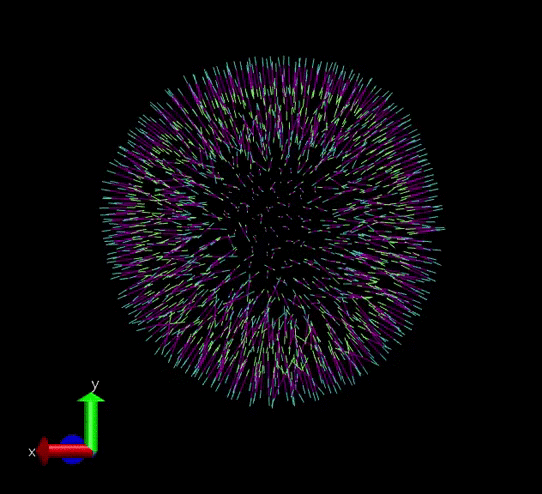A vesicle created and assigned with appropriate topology information. gif courtesy: @dexterdev

The above vesicle is made of many lipid molecules which look as below: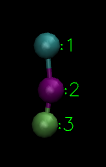A single lipid with three type of particles

The first step to create a vesicle is to decide its size. Then the next step is to decide the number of total lipids to be accomodated in the it which can be calculated based on the area per lipid calculations from literature. Once you have these information, it can be written in some easy fileformats. I choose XYZ file format as it is very straight forward. Proper resid should be assigned and for this purpose for convenience I used MATLAB scripting. You can do it in VMD /Python too. (But you know what I am lazy! ;P) As a final step you have to assign proper bond and angle topology information. This can be done via topotools.

## Writing Coordinate information to XYZ file

You can place random points on a sphere based on the algorithm mentioned in . I implemented it in MATLAB:

Ap=30^2; % projected area for 500 single leaflet
%lipids in bilayer sheet
r = 10;  % radius of the sphere
n=round((500/Ap)*4*pi*r^2);% number of points
u1=rand(1,n);
u2=rand(1,n);
lambda=acos(2.*u1-1)-(pi/2);
phi=2*pi.*u2;

xp1    = r.*cos(lambda).*cos(phi);
yp1    = r.*cos(lambda).*sin(phi);
zp1    = r.*sin(lambda);


The above code snippet places random points on a sphere with radius 10 units. The number of points are calculated according to a parameter Ap from . As we discussed in the previous article, Ap is the projected area for 1000 lipid bilayer sheet(500 on each leaflet). So for a sphere of radius 10, the surface area will be 4 * pi * 10^2 and corresponding number of lipids on a sphere of radius 10 should be n=round((500/Ap)*4*pi*r^2). These points will be the heads of the outer leaflet. Now it is easy to extend tail atoms for respective outerheads. Similarly this procedure is repeated for inner leaflet heads and corresponding tails too. Finally you write the data to a XYZ file. The whole code is available here: https://github.com/dexterdev/Molecular_Dynamics/blob/master/Vesicle_structure_coordinate/vesicle_coord.m

Now we can convert it to a PDB(protein data bank) file for convenience. You can do it as below using tcl scripting in VMD:

mol new vesicle_rad10_apl1.8.xyz
set sel [atomselect 0 all]
#select all atoms
$sel writepdb vesicle_rad10_apl1.8.pdb #write pdb file  The below MATLAB script reds the PDB file and renumbers the resid numbers properly. Resid numbers are numbers which specify each individual lipid in this example: pdb=pdbread('vesicle_rad10_apl1.8.pdb'); len=(length({pdb.Model.Atom.X}))/3; A=[1:len;1:len;1:len]; A=reshape(A,[len*3 1]); A = num2cell(A); [pdb.Model.Atom.resSeq] = A{:}; pdbwrite('vesicle_resid_renumbered_rad10_apl1.8.pdb',pdb);  That was all about coordinate information and saving it properly in a PDB file. ## Assigning a proper structure/topology file The PDB file which we have is just a bunch of points(particles). We haven't specified connections between the particles. That means the bond information is missing and since they are 3 atom lipid molecules and their angle is significant in our experiments, we have to specify angles too. Here is where topotools coming handy. You can code it using the tcl interface in VMD. Below is the script: mol addfile ./vesicle_resid_renumbered_rad10_apl1.8.pdb package require topotools package require pbctools set sel1 [atomselect 0 "sqrt(x^2+y^2+z^2)>9.9"]$sel1 set type H
#selecting atoms in the outersphere and naming it H
#outersphere is at a radius of 10

set sel2 [atomselect 0 "(sqrt(x^2+y^2+z^2)>5.7) and (sqrt(x^2+y^2+z^2)<5.9)"]
$sel2 set type H set sel3 [atomselect 0 "(sqrt(x^2+y^2+z^2)>8.9) and (sqrt(x^2+y^2+z^2)<9.1)"]$sel3 set type T1

set sel4 [atomselect 0 "(sqrt(x^2+y^2+z^2)>6.7) and (sqrt(x^2+y^2+z^2)<6.9)"]
$sel4 set type T1 set sel5 [atomselect 0 "(sqrt(x^2+y^2+z^2)>7.7) and (sqrt(x^2+y^2+z^2)<8.1)"]$sel5 set type T2

set sel6 [atomselect 0 "(sqrt(x^2+y^2+z^2)>7.9)"]
$sel6 set segname U set sel7 [atomselect 0 "(sqrt(x^2+y^2+z^2)<7.9)"]$sel7 set segname L

set blist {}
set natoms [molinfo top get numatoms]
for {set a 0; set b 1} {$b <$natoms} {set a [expr {$a + 3}]; set b [expr {$b + 3}]} {
lappend blist [list $a$b H-T1]
}

set bblist {}
set natoms [molinfo top get numatoms]
for {set c 1; set d 2} {$d <$natoms} {set c [expr {$c + 3}]; set d [expr {$d + 3}]} {
lappend bblist [list $c$d T1-T2]
}
set tlist [concat $blist$bblist]
topo setbondlist type $tlist #The above loops #specifying two kinds of bond info H-T1 and H-T2 #and then writes it to bond list set anglelist {} set natoms [molinfo top get numatoms] for {set a 0; set b 1; set c 2} {$c < $natoms} {set a [expr {$a + 3}]; set b [expr {$b + 3}]; set c [expr {$c + 3}]} {
lappend anglelist [list H-T1-T2 $a$b $c] } topo setanglelist$anglelist

#The above loop
#specifying H-T1-T2 angle info
#and then writes it to angle list

set sel [atomselect 0 all]
\$sel set mass 1

pbc set {25 25 25} -all -molid top
pbc box -center origin
#setting box dimension with pbc tool

# Finally we write the information to a data file
#which has the coordinates and topology/structure information


## Coming up in the next articles

Until now we have seen the:

And the next articles will deal with:

• Setting up custom potential via tabulated potential files in LAMMPS
• Running our vesicle using different forcefields and playing with it.

So that's for today.

All codes available in my github repository here.

## Support #STEMsocial

Are you aware of the fact that this is is the oldest STEM curation project on the blockchain? Keep enjoying the content which gets curated by #stemsocial. If you are a #STEM content creator this is the community for you. Or if you are a science enthusiast who is interested in learning more stuff and enjoys our content in blockchain, please support us and engage in communicating with us via comments and doubts. You can:

## Bye Bye friends! Keep on HIVE-ing!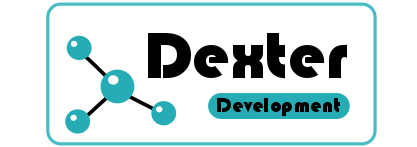credit: @mathowl

Sort:

@tipu curate :)

Upvoted 👌 (Mana: 0/20)

Congratulations @dexterdev! You have completed the following achievement on the Hive blockchain and have been rewarded with new badge(s) :You published more than 150 posts. Your next target is to reach 200 posts.

If you no longer want to receive notifications, reply to this comment with the word STOP

Do not miss the last post from @hivebuzz: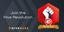Revolution! Revolution!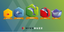HiveBuzz - Hive Gamification Experience
###### Vote for us as a witness to get one more badge and upvotes from us with more power!